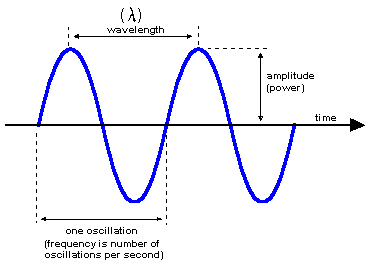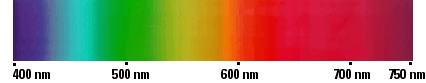# wavelength

(redirected from on the same wavelength)
Also found in: Dictionary, Thesaurus, Medical, Idioms.

## wavelength

the distance, measured in the direction of propagation, between two points of the same phase in consecutive cycles of a wave.

## Wavelength

The distance between two points on a wave which have the same value and the same rate of change of the value of a parameter, for example, electric intensity, characterizing the wave. The wavelength, usually designated by the Greek letter λ, is equal to the speed of propagation c of the wave divided by the frequency of vibration f; that is, λ = c/f (see illustration). See Wave (physics), Wave motion

## wavelength

(wayv -length) Symbol: λ. The distance over which a periodic wave motion goes through one complete cycle of oscillation, i.e. the distance traveled during one period. Thus for a sinusoidal wave motion, such as electromagnetic radiation, it is the distance between two successive peaks or troughs. For electromagnetic radiation, wavelength is related to frequency, ν, by νλ = c , where c is the speed of light. Wavelength is measured in meters or in multiples or submultiples of meters; for example, the wavelength of light is usually given in nanometers while that of infrared radiation is usually quoted in micrometers.

## wavelength

[′wāv‚leŋkth]
(physics)
The distance between two points having the same phase in two consecutive cycles of a periodic wave, along a line in the direction of propagation.

## wavelength

For light waves or sound waves, the distance between two successive points of a periodic wave in the direction of propagation, in which the oscillation has the same phase; the distance the wave travels in one period. For light waves three common units of wavelength are: micrometer, nanometer, and angstrom.

## wavelength

The distance between crests of a wave. The wavelength determines the nature of the various forms of radiant energy that comprise the electromagnetic spectrum. For electromagnetic waves, the wavelength in meters is computed by the speed of light divided by frequency (300,000,000/Hz). For sound waves, the wavelength is determined by 335/Hz. See optical bands.

Length of a WaveThe wavelength is the distance between crests. The higher the frequency, the shorter the wavelength.

Wavelengths of Visible LightThe wavelengths of light that humans can see range from approximately 400 nm to 750 nm.
Site: Follow: Share:
Open / Close